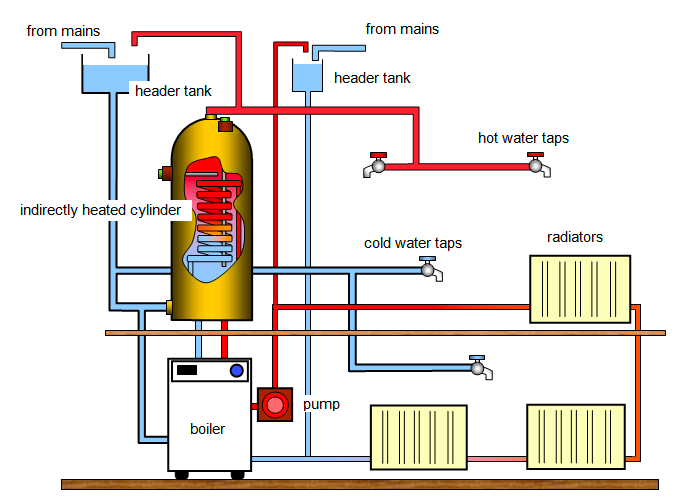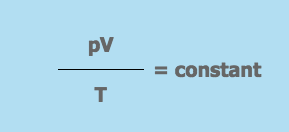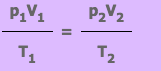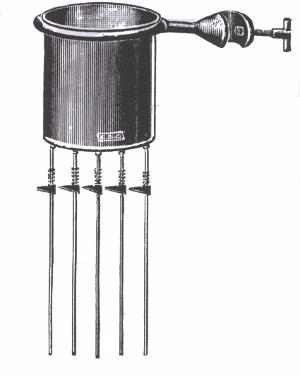# FORM THREE PHYSICS STUDY NOTES TOPIC TOPIC 5: THERMAL EXPANSION & TOPIC 6: TRANSFER OF THERMAL ENERGY

Posted on
FORM THREE PHYSICS STUDY NOTES TOPIC TOPIC 5: THERMAL EXPANSION & TOPIC 6: TRANSFER OF THERMAL ENERGYTOPIC 5: THERMAL EXPANSION
Thermal Energy
The Concept of Heat
Explain the concept of heat
Heat – Is the transfer of energy due to temperature differences.
Temperature – Is the degree of hotness or coldness of a body.
Or heat is a form of energy which raises the temperature of the substance.
SI Unit of Temperature is Kelvin (K).Conversion of centigrade into fahrerinheit given by:f = 9/5ºC + 32
Conversion of Fahrenheit into centigrade given by C = 5/9/ ( F – 32). Thermometer used for measurement of temperature. Maximum thermometer is the one which is used to measure the highest temperature obviously filled with mercury.
A Minimum thermometer is used to measure the lowest temperature and it is filled with alcohol. Combined maximum and minimum thermometer (Six’s thermometer) is used to measure highest and lowest temperature at the same time.
SI Unit of Heat is Joule (J) mathematically heat energy given by:
• H = MC (Q2 – Q1)
• M = Mass of substance
• C = Specific heat capacity
• Q= Final Temperature
• Q1 = Initial Temperature
Source of Thermal Energy in Everyday Life
State the source of thermal energy in everyday life
There are numerous sources of energy such as the sun, fuels, nuclear sources, geothermal, electricity among others. The most important source of thermal energy is the sun. The sun generates its energy by a process called thermonuclear fusion. Most sources of thermal energy derive their energy from the sun. Thermal energy from the sun makes life on earth possible.
Difference between Heat and Temperature
Distinguish between heat and temperature
Heatandtemperatureare related and often confused. More heat usually means a higher temperature.
Heat(symbol:Q) is energy. It is the total amount of energy (both kinetic and potential) possessed by the molecules in a piece of matter. Heat is measured in Joules.
Temperature(symbol:T) is not energy. It relates to the average (kinetic) energy of microscopic motions of a single particle in the system per degree of freedom. It is measured inKelvin (K), Celsius (C) or Fahrenheit (F).
When you heat a substance, either of two things can happen: the temperature of the substance can rise or thestateof substance can change.
Heat Temperature
Definition Heat is energy that is transferred from one body to another as the result of a difference in temperature. Temperature is a measure of hotness or coldness expressed in terms of any of several arbitrary scales like Celsius and Fahrenheit.
Symbol Q T
Unit Joules Kelvin, Celsius or Fahrenheit
SI unit Joule Kelvin
Particles Heat is a measure of how many atoms there are in a substance multiplied by how much energy each atom possesses. Temperature is related to how fast the atoms within a substance are moving. The ‘temperature’ of an object is like the water level – it determines the direction in which ‘heat’ will flow.
Ability to do work Heat has the ability to do work. Temperature can only be used to measure the degree of hea
The Concept of Thermal Expansion of Gases
Explain the concept of thermal expansion of gases
Gases expand when heated just like solids and liquids. This is because the average kinetic energy of the molecules in a gas is directly proportional to the absolute temperature of the gas. Heating the gas increases the kinetic energy of its molecules, making them vibrate more vigorously and occupy more space.
The Relationship between Volume and Temperature of Fixed Mass of Air at Constant Pressure
Investigate the relationship between volume and temperature of fixed mass of air at constant pressure
Three properties are important when studying the expansion of gases. These are; pressure, volume and temperature. Charles law states that the volume of a fixed mass of gas is directly proportional to the absolute (Kelvin) temperature provided the pressure remains constant. Mathematically V1T2 = V2T1.
Example 2
the volume of gas at the start is recorded as 30 cm3with a temperature of 30°C. The cylinder is heated further till the thermometer records 60°C. What is the volume of gas?
Solution:
We know,V/T = constant
therefore,
V1/T1=V2/T2
V1 =30 cm3
T1 =30°C = 30+273 = 303K(remember to convert from Celsius to Kelvin)
T2 =60°C = 60+273 = 333K
V2 =?
V1/T1=V2/T2
V2=V1xT2/T1
V2=30×333/303
= 32.97 cm3
The Relationship between Pressure and Volume of a Fixed Mass of Air at Constant Temperature
Investigate the relationship between pressure and volume of a fixed mass of air at constant temperature
The relationship obtained when the temperature of a gas is held constant while the volume and pressure are varied is known as Boyle’s law. Mathematically, P1V1 = P2V2. Boyle’s law states that the volume of a fixed mass of gas is inversely proportional to its pressure if the temperature is kept constant.
PressurexVolume = constant
pxV = constant
Example 3
The volume of gas at the start is 50 cm3with a pressure of 1.2 x 105Pascals. The piston is pushed slowly into the syringe until the pressure on the gauge reads 2.0 x 105Pascals. What is the volume of gas?
Solution:
We know
p x V = constant
therefore,
p1xV1= p2xV2
p1=1.2 x 105Pascals
V1=50 cm3
p2=2.0 x 105Pascals
V2=?
p1xV1= p2xV2
V2=p1xV1/p2
V2=1.2×105x50/2.0 x 105
V2= 30 cm3
The Relationship between Pressure and Temperature of a Fixed Mass of Air at Constant Volume
Investigate the relationship between pressure and temperature of a fixed mass of air at constant volume
To investigate the relationship between the pressure and the temperature of a fixed mass, the volume of the gas is kept constant. The pressure is then measured as the temperature is varied. P1/T1 = P2/T2 ,this is called pressure law. The pressure law states that the pressure of a fixed mass of a gas is directly proportional to the absolute temperature if the volume is kept constant
Example 4
Pressure of gas is recorded as 1.0 x 105N/m2at a temperature of 0°C. The cylinder is heated further till the thermometer records 150°C. What is the pressure of the gas?
Solution:
We know,p/T = constant
therefore,
p1/T1= p2/T2
p1=1.0 x 105N/m2
T1=0°C = 0+273 = 273K(remember to convert from Celsius to Kelvin)
T2=150°C = 150+273 = 423K
p2 =?
p1/T1= p2/T2
p2=p1xT2/T1
p2=1.0×105x423/273
= 1.54 x 105N/m2
The General Gas Equation from the Gas Laws
Identify the general gas equation from the gas laws
The three gas laws give the following equations:
1. pV = constant(when T is kept constant)
2. V/T = constant(when p is kept constant)
3. P/T= constant(when V is kept constant)
These 3 equations are combined to give the ideal gas equation:Where,
• p = the pressure of the gas
• V = the volume the gas occupies
• T = the gas temperature on the Kelvin scale
From this equation we know that if a fix mass of gas has starting values of p1, V1 and T1, and then some time later has value p2, V2 and T2, the equation can be written as:Exercise 1
Sabah pumps up her front bicycle tyre to 1.7 x 105Pa. The volume of air in the tyre at this pressure is 300 cm3. She takes her bike for a long ride during which the temperature of the air in the tyre increases from 20°C to 30°C. Calculate the new front tyre pressure assuming the tyre had no leaks and so the volume remained constant?
Absolute Scale of Temperature
Explain absolute scale of temperature
Absolute zero is the lowest temperature that can be attained theoretically. It is not possible to attain this temperature because all gases liquefy before attaining it. The kelvin scale of temperature is obtained by shifting the vertical axis to -273 degrees Celsius and renaming it 0 K. On the scale 0 degrees Celsius becomes 273 K and 100 degrees Celsius corresponds with 373 K.
Convertion of Temperature in Degrees Centigrade (Celsius) to Kelvin
Convert temperature in degrees centigrade (celsius) to kelvin
The Kelvin temperature scale takes its name after Lord Kelvin who developed it in the mid 1800s. It takes absolute zero as the starting point and temperature measurements are given the symbol K (which stands for “Kelvin”). Temperature differences on the Kelvin scale are no different to those on the Celsius (°C) scale. The two scales differ in their starting points. Thus, 0°C is 273K.
Converting from Celsius to Kelvin
• Temperature in °C + 273 = Temperature in K
Converting from Kelvin to Celsius
• Temperature in K – 273 = Temperature in °C
Example 5
The temperature of a gas is 65 degrees Celsius. Change it to the kelvin scale.
Solution
T(K) = degrees Celsius + 273, T(K) = 65+273
therefore T(K) = 338 K.
Standard Temperature and Pressure (S.T.P)
Explain standard temperature and pressure (S.T.P)
The standard temperature and pressure (S.T.P) is a set of conditions for experimental measurements to enable comparisons to be made between sets of data. The standard temperature is 0 degrees Celsius (273 K) while the standard pressure is 1 atmosphere (101300 Pa or 760 mm of mercury).
Expansion of Gas in Daily Life
Apply expansion of gas in daily life
Land and sea breezes are the result of expansion of air caused by unequal heating and cooling of adjacent land and sea surfaces. The piston engine and firing bullets from guns work under principles of expansion of gases.
TOPIC 6: TRANSFER OF THERMAL ENERGY
Conduction
The Concept of Conduction of Heat
Explain the concept of conduction of Heat
Conduction is the transfer of heat energy through solids, for example, metals. Generally solid substances contain particles which are close together. Each particle vibrates at one position but cannot move to another position.
Solid materials differ greatly in their ability to conduct HEAT.
Good and Bad Conductors of Heat
Identify good and bad Conductors of Heat
Solid materials differ greatly in their ability to conduct HEAT.
Good conductors
These are the substances which allows the passage of heat energy easily example all metals.
Metals contain tiny particles called electrons (particles that carry electricity through metals) which are free to move inside the metal and carry energy from hotter places to colder places.
These are materials which does not allow the passage of heat and electricity e.g Non – metals, woods.
 GOOD CONDUCTOR BAD CONDUCTOR Silver Aluminum Brass Iron Copper Steel Glass Wood Asbestos Water Air
How to Minimize Heat Losses due to Conduction
Explain how to minimise Heat losses due to Conduction
There are some simple ways to reduce heat loss, including fitting carpets, curtains and draught excluders.
Heat loss through windows can be reduced using double glazing. The gap between the two panes of glass is filled with air. Heat loss through conduction is reduced, as air is a poor conductor of heat. Heat transfer by convection currents is also reduced by making the gap is very narrow.
Heat loss through walls can be reduced using cavity wall insulation. This involves blowing insulating an material into the gap between the brick and the inside wall, which reduces the heat loss by conduction. The material also prevents air circulating inside the cavity, therefore reducing heat loss by convection.
Knowledge of Conduction in Daily Life
Apply knowledge of conduction in daily life
The difference in conductivity of various materials can be demonstrated using Edser’s apparatusThe apparatus consists of copper can with identical rods of aluminum, copper, lead and iron fixed to the bottom of the can.
The can is supported by a metal ring which is clamped to a retort stand. When hot water is poured inside the copper can, heat will be passed along the rods by conduction.
After some time, it will be observed that wax coatedon the rods will melt and move down the rods. Note how far along the rods the wax has melted when the apparatus reaches a steady state.
This indicates that the materials from which the rods are made have different thermal conductivities. Of the four metal rods, the copper rod is observed to conduct heat more quickly than the rest.
Conduction of Heat Energy through Liquids
• All liquids expect mercury and gases are poor conductors of heat.
• Gases are far worse conductors of heat than liquids.
• Fluids are bad conductors of heat. They transfer heat by means of convection.